### 1．小星星

N=5

x = r*sin(nθ)*cos(θ)

y = r*sin(nθ)*sin(θ)      （0≤θ≤2π）

<!DOCTYPE html>

<title>小星星</title>

<script type=”text/javascript”>

function draw(id)

{

var canvas=document.getElementById(id);

if (canvas==null)

return false;

var context=canvas.getContext(‘2d’);

context.fillStyle=”#EEEEFF”;

context.fillRect(0,0,400,300);

context.strokeStyle=”blue”;

context.lineWidth=2;

context.beginPath();

var r=120;                    // 下面可进行修改的语句 （3）

var n=5;                      // 下面可进行修改的语句 （3）

for (theta=0;theta<=2*Math.PI;theta+=Math.PI/64)

{

b=r*Math.sin(n*theta);    // 下面可进行修改的语句 （3）

x=200+b*Math.cos(theta);

y=150+b*Math.sin(theta);

if (theta==0)

context.moveTo(x,y);

else

context.lineTo(x,y);

}

context.stroke();

}

</script>

<canvas id=”myCanvas” width=”400″ height=”300″></canvas>

</body>

</html>

将上述HTML代码保存到一个html文本文件中，再在浏览器中打开包含这段HTML代码的html文件，可以看到在浏览器窗口中绘制出如图1所示的5瓣花卉图案。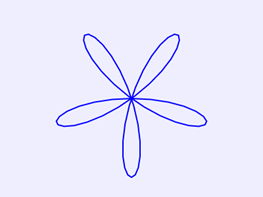我们将上面程序中标识的语句1“var r=120;”修改为“var r=50;”，适当减小初始半径，使得绘制的图案不会超出画布的范围，在将标识的语句3“b=r*Math.sin(n*theta);”修改为“b=r*(1+Math.sin(n*theta)/2);”，则在画布中绘制出如图2所示的5瓣花卉图案。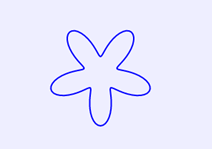这个图案比图1中的图案的感觉是中间向外扩展了，使得中心部位出现空白。再次修改语句3为“b=r*(2+Math.sin(n*theta)/2);”，以增大中间部分的空白，则在画布中绘制出如图3所示的图案，像小星星吗？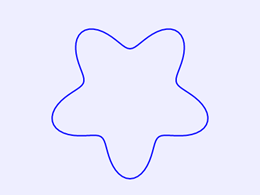上面程序中的n值也可修改，例如在上面绘制小星星图案的程序中，将标识语句2

“var n=5;”修改为“var n=6;”，则在画布中绘制出如图4所示的图案。像六角雪花图案吗？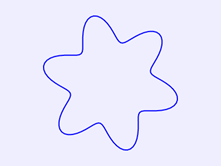我们这样想一想，若将n的值修改为一个实数（有小数部分）会这样呢？例如，将

“var n=5;”修改为“n=5.05;”，则在画布中绘制出如图5所示的图案。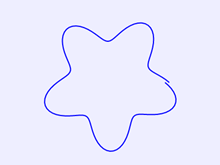### 2．环带

由图5知，θ值取0~2π时，正好绘制一圈，由于n取非整数时，曲线不封闭，因此，若取θ值为0~10π，绘制5圈，可得到一个环带图案。为读者引用和下面讲述方便，给出完整HTML文件内容如下。

<!DOCTYPE html>

<title>环带</title>

<script type=”text/javascript”>

function draw(id)

{

var canvas=document.getElementById(id);

if (canvas==null)

return false;

var context=canvas.getContext(‘2d’);

context.fillStyle=”#EEEEFF”;

context.fillRect(0,0,400,300);

context.strokeStyle=”red”;

context.lineWidth=2;

context.beginPath();

var n=5.05;

k=10;

for (theta=0;theta<=k*Math.PI;theta+=Math.PI/120)

{

var r=110*(1+Math.cos(n*theta)/5);

var x =200+ r*Math.cos(theta);

var y =150+ r*Math.sin(theta);

if (theta==0)

context.moveTo(x,y);

else

context.lineTo(x,y);

}

context.stroke();

}

</script>

<canvas id=”myCanvas” width=”400″ height=”300″></canvas>

</body>

</html>

在浏览器中打开包含这段HTML代码的html文件，可以看到在画布中绘制的五角环带，如图6所示。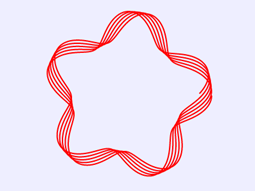将上面程序中的n值由“n=5.05”修改为“n=9.05”，则在画布中绘制出如图7所示的环带。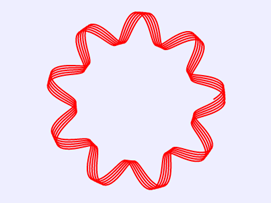若将上面程序中的n值修改为9.2，且将k值修改为12，则在画布中绘制出如图8所示的圆环带。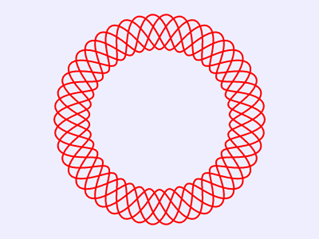若将上面程序中的n值修改为5.5，且将k值修改为12，则在画布中绘制出如图9所示的圆环。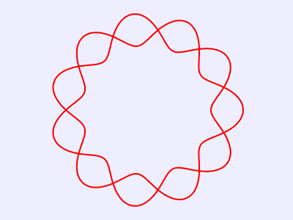在绘制图9的程序中，再将语句“var r=110*(1+Math.cos(n*theta)/5);”修改为

“var r=90*(1+Math.cos(n*theta)/2);”则在画布中绘制出如图10所示的图案。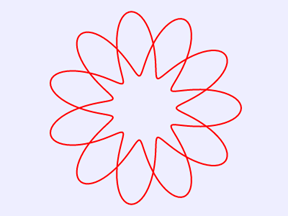1.《JavaScript图形实例：从小星星到环带》援引自互联网，旨在传递更多网络信息知识，仅代表作者本人观点，与本网站无关，侵删请联系页脚下方联系方式。

2.《JavaScript图形实例：从小星星到环带》仅供读者参考，本网站未对该内容进行证实，对其原创性、真实性、完整性、及时性不作任何保证。

3.文章转载时请保留本站内容来源地址，https://www.cxvn.com/study/26691.html